# Modelling & Numerical Investigation of the Effectiveness of Plate Heat Exchanger for Cooling Engine Oil Using ANSYS CFX

Modelling & Numerical Investigation of the Effectiveness of Plate Heat Exchanger for Cooling Engine Oil Using ANSYS CFX
Department of Mechanical Engineering, FET, University of Botswana, Gaborone, P. Bag 0061, Botswana

Corresponding Author Email:
agarwala@ub.ac.bw
Page:
653-658
|
DOI:
https://doi.org/10.18280/ijht.390237
23 July 2020
|
Accepted:
12 February 2021
|
Published:
30 April 2021
| Citation

OPEN ACCESS

Abstract:

Heat exchangers are used for various industrial application for transfer of enthalpy from hot fluid to cold. One of them is Plate Heat Exchanger which finds its application in evaporating systems. The compactness, high effectiveness and easy maintenance of Plate Heat Exchanger makes it best choice for process industries. The current research investigates the application of Plate Heat Exchanger in cooling of engine oil using techniques of Computational Fluid Dynamics for low, medium and high Reynolds number using ANSYS CFX software. The CAD model is developed using Creo design software and turbulence model used for analysis is RNG k-epsilon which gives good predictions for complex flows involving swirls. The CFD analysis is conducted for different values of Reynolds number. The temperature distribution, effectiveness and overall heat transfer coefficient is determined for different values of Reynolds number.

Keywords:

conductivity, plate heat exchanger, CFD, thermo-physical properties, Reynolds number, effectiveness

1. Introduction

The first commercial application of Plate Heat Exchanger (PHE) commenced in 1929 by food industry . The PHE comprises of gasketed or brazed series of individual plates (chevron). These series of plates are pressed between covers and the gaps between plates are nearly 1.2mm to 1.5mm.

The geometric characteristics of chevron type plates used in Plate Heat Exchanger is shown in Figure 1. Here Lw is plate width, β is the corrugation angle, Dp is port dimeter, Lh is horizontal port distance.

## 1.jpg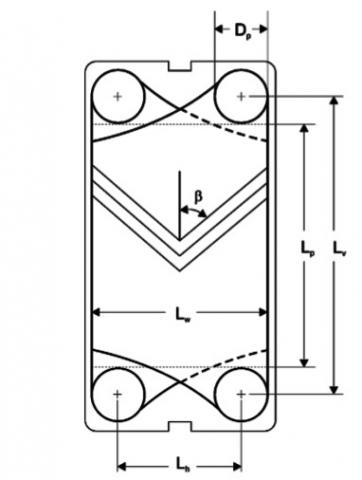Figure 1. Geometric characteristics of chevron plate 

Sarraf et al.  investigated the condensation process on PHE using experimental techniques. The working fluid taken for experimentation was pentane. The characterization of heat transfer was done using infrared technique to study the effect of superheated vapor on flow distribution on flow distribution.

Gradeck et al.  Conducted experimental studies to investigate hydrodynamic behavior of fluid flow through corrugated channel. The tests were conducted for both laminar flow regime and turbulent flow regime. The findings have shown that thermal boundary layer is destabilized resulting in augmentation of heat transfer.

Bobbili et al.  conducted experimental investigation the thermo-hydraulic performance of PHE by varying number of channels. The plate package considered for analysis were 21 and 81. The results have shown that flow maldistribution can occur even for smaller plate package with reduction of inlet/outlet cross sectional area. The plate package has significant effect on velocity and pressure distribution.

Warnakulasuriya and Worek  conducted experimental investigation on commercial PHE absorbent salt solution. The experimental findings have shown that higher HTC are achieved for smaller flow paths and thereby the overall size of PHE can be kept minimum with low fouling rates.

Kumar et al.  conducted experimental investigation on plate heat exchanger using various nanoparticles like ZnO, TiO2 with water as base fluid. The results have shown a significant increase in HTC (nearly 53%) with nominal rise in pressure drop.

Various studies [8, 9] in the past employed CFD simulation to evaluate the temperature distribution, heat transfer performance and pressure drop of plate heat exchanger.

Focke et al. [10, 11] investigated the effect of corrugation angle (β) on thermo-hydraulic performance of PHE using experimental technique. The findings have shown that β has significant effect on flow distribution, vortex generation which ultimately augments or reduces HTC.

Muley et al. [12, 13] investigated the effect of variables β and γ on thermo-hydraulic performance of PHE. The research findings have shown that with increase of β or γ, the Nusselt number and friction factor increased.

Lee, J. and Lee, K.S. [14, 15] conducted CFD analysis on chevron type PHE and optimized its design to improve its thermal and fluid flow characteristics.

The current research investigates the effectiveness of PHE for cooling engine oil using CFD. The analysis is conducted for low, medium and high Reynolds number. The hot fluid used in numerical analysis is engine oil and cold fluid used is water. The turbulence model used for CFD analysis is RNG k-Epsilon .

2. Material and Methods

The numerical analysis of PHE is conducted using techniques of Computational Fluid Dynamics (CFD). The CFD is governed by Navier’s Stokes equation which is based on conservation of energy, mass and momentum conservation. The CAD model of PHE is developed using extrude, pattern tool of Creo design package as per dimensions given in Table 1 below.

Table 1. Dimensions of PHE

 Particular Dimensions Height of plate 10cm Width of plate 5cm Gap between plates 1mm Thickness 7mm Number of hot chambers 3 Number of cold chambers 3

The CAD model is then converted in IGES format and exported in ANSYS design modeler as shown in Figure 2 representing different parts of plate heat exchanger. The IGES format file is imported in ANSYS design modeler where it is checked for geometric errors, hard edges.

The imported CAD model is meshed with hexahedral elements with fine sizing and curvature. The mesh generated is of hexahedral shape and structured for all six plates while for remaining portion of PHE i.e. neat inlet and outlet tubes of hot and cold fluid has unstructured mesh as shown in Figure 3 below. The number of elements generated is 44,720 and number of nodes generated is 57,802.

The model is applied with fluid inlet and outlet boundary conditions. The thermophysical properties of water and engine oil is shown in Table 2 and Table 3 below.

Table 2. Water properties 

 Properties Values Density 1025 Kg/m3 Specific heat 4007 J/Kg·K Viscosity .00108 Ns/m2 Thermal conductivity .596 W/m-K

Table 3. Engine oil properties 

 Properties Values Density 8352 g/cm3 Specific heat 2901 J/Kg·K viscosity .014588 Ns/m2 Thermal conductivity .149 W/m-K

The left side tube geometry as shown in Figure 4 is defined with engine oil (hot fluid) domain and right-side tube geometry as shown in Figure 5 is defined with water (cold fluid) domain.

The fluid inlet mass flow rate is applied at water inlet and engine oil inlet for low Reynolds number, medium Reynolds number and high Reynolds number. The reference pressure is defined at 1 atm for both the fluids. The solver setting is set to RMS residual value of 1e-4 and 200 iterations are carried out in solution stage. The equations motions are formulated for each discretized elements of computational domain which forms a system of algebraic equations. These algebraic equations are solved numerically .

## 2.png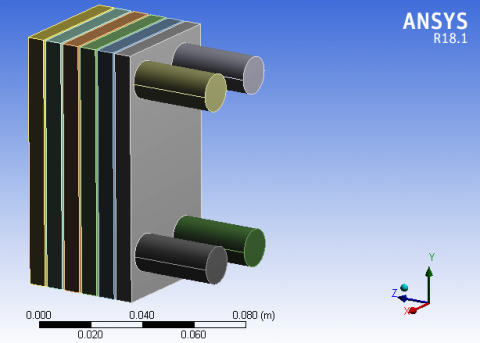Figure 2. Different parts of plate heat exchanger

## 3.png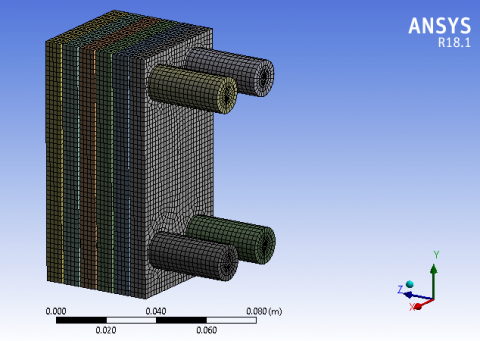Figure 3. Meshed model of plate heat exchanger

## 4.png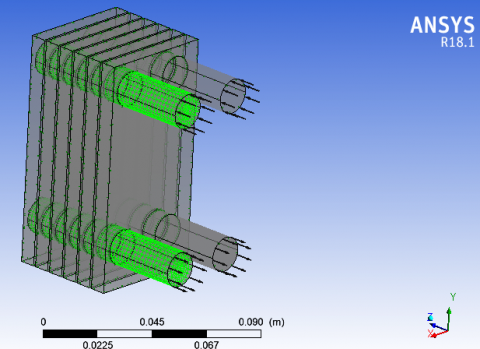Figure 4. Engine oil (hot fluid) domain

## 5.png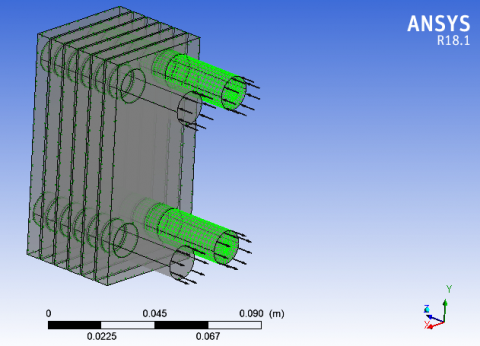Figure 5. Water (cold fluid) domain

2.1 Data reduction

For constant specific heats with no change of phase, the heat transfer of cold fluid is given by Qc and for hot fluid is given by Qh .

Qc = (m Cp) c (Tc2 − Tc1)        (1)

And

Qh = (m Cp) h (Th1 − Th2)        (2)

Now from energy conservation we know that:

Qc = Qh = Q

The log mean temperature difference (LMTD) is given by the relation :

$\mathrm{LMTD}=\frac{\left(T_{1}-t_{2}\right)-\left(T_{2}-t_{1}\right)}{\ln \frac{\left(T_{1}-t_{2}\right)}{\left(T_{2}-t_{1}\right)}}$  (3)

With the assumed Uo, an value of the heat total heat transfer area of plate required can be calculated as given by relation.

Q = Uo At ∆TLM Ft    (4)

where,

Uo = overall heat transfer coefficient in (W/(m²·c)),

Ft = log. Mean correction factor,

∆TLM = log Mean temp. difference,

hm = convective heat transfer coefficient,

hw =convective heat transfer coefficient for water,

tp = thickness of plate of plate,

K= Thermal conductivity of material,

The Nusselt number is defined as,

$\mathrm{Nu}=\frac{h D}{K}$   (5)

where, h is heat transfer coefficient and D is hydraulic diameter. K is thermal conductivity.

3. Results and Discussion

The CFD analysis  is conducted on compact heat exchanger for low Reynolds number, medium Reynolds number and high Reynolds number. The temperature variation and heat transfer coefficient are determined from CFD simulation. The hot side temperature plot is shown in Figure 6 for low Reynolds number.

The plot shows higher temperature near fluid inlet region and on 1st and 2nd hot plate. The higher temperature zone for 1st hot plate is bigger than on 2nd hot plate. The cold side temperature plot is shown in Figure 7.

The plot shows lower temperature near fluid inlet as shown by blue colored region. The fluid becomes hotter as it reaches 2nd plate.

For medium Reynolds number the zone of high temperature is observed in 3rd plate also as shown in Figure 8. The temperature is higher near the hot fluid inlet tube as shown by red colored region ranging from 356 K to 362 K. The cold side temperature plot is shown in Figure 9 for medium Reynolds number.

## 6.jpg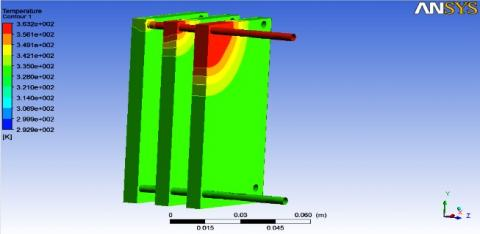Figure 6. Engine oil (hot fluid) temperature for low Reynolds number

## 7.jpg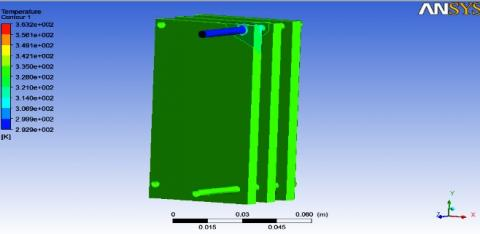Figure 7. Sea water (cold fluid) side temperature for low Reynolds number

## 8.jpg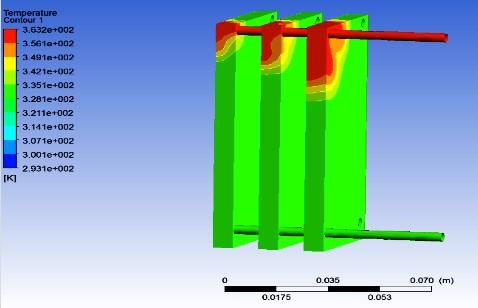Figure 8. Engine oil (hot fluid) temperature for medium Reynolds number

## 9.jpg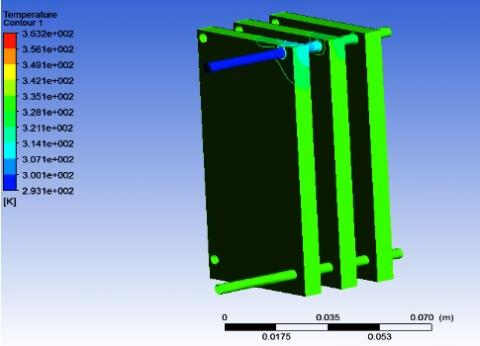Figure 9. Sea water (cold fluid) side temperature for high Reynolds number

The plot shows lower temperature near fluid inlet as shown by blue colored region. The temperature is higher in 2nd plate near cold fluid inlet tube for high Reynolds number as compared to low Reynolds number. For high Reynolds number the temperature of 3rd plate is 356 K represented by dark orange color as shown in Figure 10.

The temperature on 3rd plate is lower in high Reynolds number as compared to medium Reynolds number (Figure 8). Similarly, for cold fluid, the temperature on 2nd plate (Figure 11) is higher as compared to medium Reynolds number.

## 10.jpg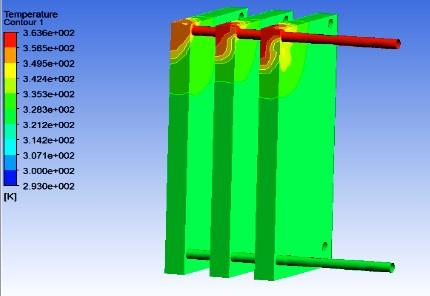Figure 10. Engine oil (hot fluid) temperature for high Reynolds number

## 11.jpg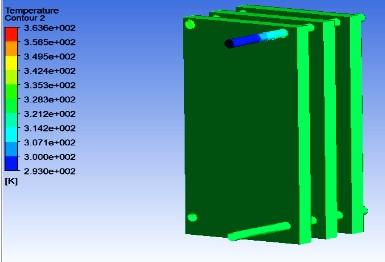Figure 11. Sea water (cold fluid) side temperature for high Reynolds number

The fluid flow path for hot and cold fluids is shown in Figure 12 below. The red streamlines represent fluid flow of engine oil (hot fluid) while dark blue lines represent fluid flow for water (cold fluid).

## 12.jpg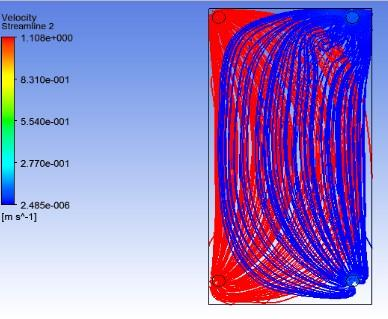Figure 12. Fluid flow path for hot and cold fluids

The heat transfer rate is determined from CFD analysis. The Nusselt number is then calculated from heat transfer rate using Eq. 5. The HTC values cold and hot fluid obtained from CFD analysis is shown in Table 4 and Table 5 respectively.

Table 4. HTC (cold fluid) and Nusselt number for low Reynold number

 Reynolds number AVG HTC (cold fluid) Nu (cold fluid) 752 229.751 1.53 1171 297.312 1.98 1602 358.833 2.4 2040 415.874 2.78 2486 469.265 3.13

Table 5. HTC (hot fluid) and Nusselt number for low Reynold number

 Reynolds number AVG HTC (hot fluid) Nu (hot fluid) 464 140.499 3.75 492 145.02 3.85 520 149.521 4.02 807 192.375 5.17 1102 231.471 6.23

It can be observed from Figure 13, that Nusselt number increases with increase in Reynolds number for water.

The minimum Nusselt number is 1.53 for Reynolds number 752 and maximum Nusselt number is 3.13 for 2486 Reynolds number. Similarly, Nusselt number increases with increase in Reynolds number for engine oil also as shown in Figure 14.

The minimum Nusselt number is 3.75 for Reynolds number 464 and maximum Nusselt number is 6.23 for Reynolds number 1102.

## 13.jpg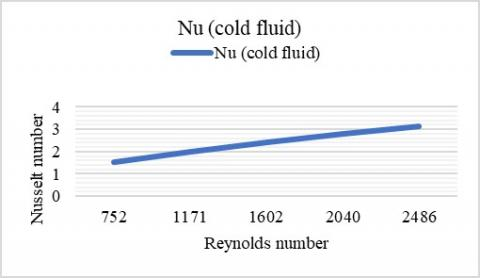Figure 13. Nusselt number curve of water for low Reynolds number

## 14.png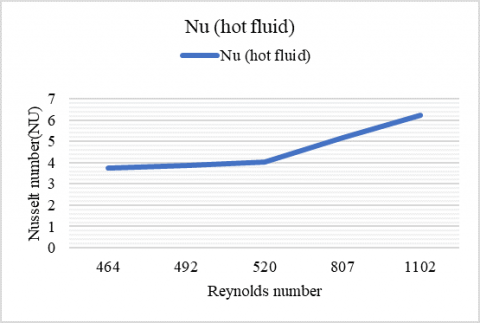Figure 14. Nusselt number curve of engine oil for low Reynolds number

The HTC values of cold and hot fluid obtained from CFD analysis for medium Reynolds number is shown in Table 6 and Table 7 respectively.

Table 6. HTC (cold) and Nusselt number for medium Reynold number

 Reynolds number AVG HTC (cold fluid) Nu (cold fluid) 9079 1035.92 6.97 18764 1577.31 10.58 28491 1982.34 13.34 37119 2862.86 19.21 46439 3730.25 25.08

Table 7. HTC (hot fluid) and Nusselt number for medium Reynold number

 Reynolds number AVG HTC (hot fluid) Nu (hot fluid) 6321 680.294 18.25 13198 1062.19 28.52 20160 1355.18 36.36 26415 1964.96 52.75 32437 2551.12 68.46

As can be observed from Figure 15, the Nusselt number increases with increase in Reynolds number for water.

The minimum Nusselt number is 6.97 for Reynolds number 9079 and maximum Nusselt number is 25.08 for 46439 Reynolds number. Similarly, Nusselt number increased with increase in Reynolds number for engine oil also as shown in Figure 16.

The minimum Nusselt number is 18.25 for Reynolds number 6321 and maximum Nusselt number is 68.46 for Reynolds number 32437.

The HTC values of cold and hot fluid obtained from CFD analysis for high Reynolds number is shown in Table 8 and Table 9 respectively.

Table 8. HTC (cold fluid) for high Reynold number

 Reynolds number AVG HTC (cold fluid) Nu (cold fluid) 55858 4143.97 27.81 65652 4314.61 28.95 74697 4953.96 33.24 84117 5352.12 35.92 93538 5746.54 38.56

Table 9. HTC (hot fluid) for high Reynold number

 Reynolds number AVG HTC (hot fluid) Nu (hot fluid) 39043 2817.56 75.63 45901 2921.02 78.41 52251 3328.86 89.36 58854 3580.01 96.12 65457 3829.52 102.8

## 15.png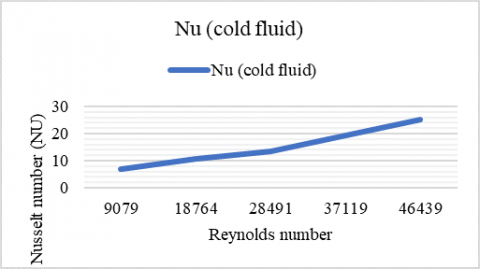Figure 15. Nusselt number curve of water for medium Reynolds number

## 16.png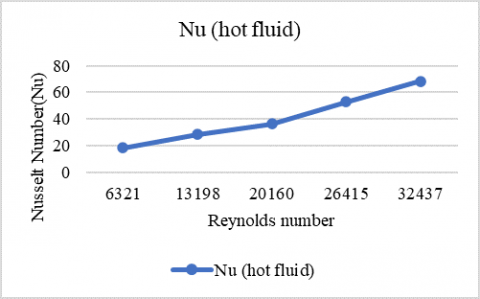Figure 16. Nusselt number curve of engine oil for medium Reynolds number

## 17.png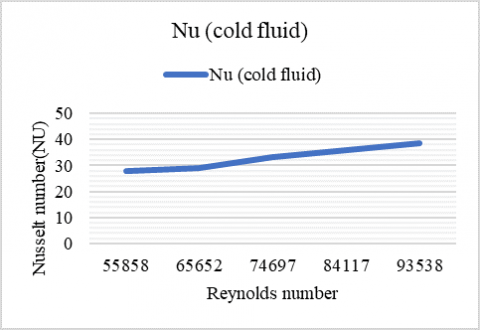Figure 17. Nusselt number curve of water for high Reynolds number

## 18.png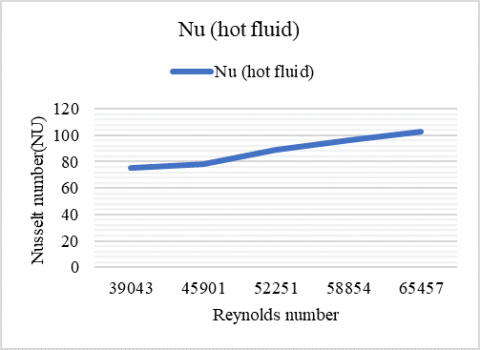Figure 18. Nusselt number curve of engine oil for high Reynolds number

Figure 17 shows that, the Nusselt number increases with increase in Reynolds number for water. The minimum Nusselt number is 27.81 for Reynolds number 55858 and maximum Nusselt number is 38.56 for 93538 Reynolds number.

Similarly, Nusselt number increases with increase in Reynolds number for engine oil also as shown in Figure 18. The minimum Nusselt number is 75.63 for Reynolds number 39043 and maximum Nusselt number is 102.8 for Reynolds number 65457.

4. Conclusion

The CFD analysis is conducted on PHE using ANSYS CFX which enabled to determine fluid flow prediction and HTC of hot and cold fluid. The application of CFD in numerical investigation of PHE shows the efficacy of tool in effective design and analysis of plate heat exchangers. The RNG k-Epsilon turbulence model gave reasonably good predictions for fluid flow behavior. The findings have shown an augmentation of heat transfer rate with increase in Reynolds number for both cold and hot fluids. It indicates the higher turbulent flow for higher Reynolds number which results into higher heat transfer rates.

The effectiveness is determined and discussed below.

1. The effectiveness of PHE for low Reynolds number is .37
2. The effectiveness of PHE for medium Reynolds number is .51
3. The effectiveness of PHE for high Reynolds number is .60

The numerical analysis based on different types of turbulence models require investigation to predict fluid flow behavior and HTC. The corrugation geometry of PHE can be optimized to achieve higher HTC without much increase on pumping power requirements.

References

 Manglik, R.M., Sundén, B., Wang, L. (2007). Plate Heat Exchangers: Design, Applications and Performance. WIT Press, Boston, ISBN: 9781853127373.

 Khan, T.S., Khan, M.S., Chyu, M.C., Ayub, Z.H. (2010). Experimental investigation of single phase convective heat transfer coefficient in a corrugated plate heat exchanger for multiple plate configurations. Applied Thermal Engineering, 30(8-9): 1058-1065. https://doi.org/10.1016/j.applthermaleng.2010.01.021

 Sarraf, K., Launay, S., Tadrist, L. (2016). Analysis of enhanced vapor desuperheating during condensation inside a plate heat exchanger. International Journal of Thermal Science, 105: 96-108. https://doi.org/10.1016/j.ijthermalsci.2016.03.001

 Gradeck, M., Hoareau, B., Lebouché, M. (2005). Local analysis of heat transfer inside corrugated channel. International Journal of Heat and Mass Transfer, 48(10): 1909-1915. https://doi.org/10.1016/j.ijheatmasstransfer.2004.12.026

 Bobbili, P.R., Sunden, B., Das, S.K. (2006). An experimental investigation of the port flow maldistribution in small and large plate package heat exchangers. Applied Thermal Engineering, 26(16): 1919-1926. https://doi.org/10.1016/j.applthermaleng.2006.01.015

 Warnakulasuriya, F.S.K., Worek, W.M. (2008). Heat transfer and pressure drop properties of high viscous solutions in plate heat exchangers. International Journal of Heat and Mass Transfer, 51(1-2): 52-67. https://doi.org/10.1016/j.ijheatmasstransfer.2007.04.054

 Kumar, V., Tiwari, A.K., Ghosh, S.K. (2016). Effect of variable spacing on performance of plate heat exchanger using nanofluids. Energy, 114: 1107-1119. https://doi.org/10.1016/j.energy.2016.08.091

 Gullapalli, V.S., Sundén, B. (2014). CFD simulation of heat transfer and pressure drop in compact brazed plate heat exchangers. Heat Transfer Engineering, 35(4): 358-366. https://doi.org/10.1080/01457632.2013.828557

 Tsai, Y.C., Liu, F.B., Shen, P.T. (2009). Investigations of the pressure drop and flow distribution in a chevron-type plate heat exchanger. International Communication in Heat Mass Transfer, 36(6): 574-578. https://doi.org/10.1016/j.icheatmasstransfer.2009.03.013

 Focke, W.W., Zachariades, J., Olivier, I. (1995). The effect of the corrugation inclination angle on the thermohydraulic performance of plate heat exchangers. International Journal of Heat and Mass Transfer, 28(8): 1469-1479. https://doi.org/10.1016/0017-9310(85)90249-2

 Focke, W.W. (1983). Turbulent convective transfer in plate heat exchangers. International Communication in Heat and Mass Transfer, 10(3): 201-210. https://doi.org/10.1016/0735-1933(83)90005-2

 Muley, A., Manglik, R.M., Metwally, H.M. (1999). Enhanced heat transfer characteristics of viscous liquid flows in a chevron plate heat exchanger. Journal of Heat Transfer, 121(4): 1011-1017. https:/doi.org/10.1115/1.2826051

 Muley, A., Manglik, R.M. (1997). Enhanced heat transfer characteristics of single-phase flows in a plate heat exchanger with mixed chevron plates. Journal of Enhanced Heat Transfer, 4(3): 187-201. https://doi.org/10.1615/jenhheattransf.v4.i3.30

 Lee, J., Lee, K.S. (2015). Friction and Colburn factor correlations and shape optimization of chevron-type plate heat exchangers. Applied Thermal Engineering, 89: 62-69. https://doi.org/10.1016/j.applthermaleng.2015.05.080

 Lee, J., Lee, K.S. (2014). Flow characteristics and thermal performance in chevron type plate heat exchangers. International Journal of Heat and Mass Transfer, 78: 699-706. https://doi.org/10.1016/j.ijheatmasstransfer.2014.07.033

 Agarwal, A., Mthembu, L. (2020). CFD analysis of conical diffuser under swirl flow inlet conditions using turbulence models. Matereials Today Proceedings, 27(2): 1350-1355. https://doi.org/10.1016/j.matpr.2020.02.621

 Sethi, C.K. (2017). CFD Analysis on effectiveness of a plate type heat exchanger using sea water and engine oil. International Journal of Advanced Mechanical Engineering, 12(1): 191-198. https://www.ripublication.com/ijame-spl/ijamev8n1spl_21.pdf.

 Jameson, A., Martinelli, l., Vassberg, J.C. (2002). Using computational fluid dynamics for aerodynamics - a critical assessment. 23rd International Congress of Aeronautical Sciences, Toronto, Canada.

 Sharif, A., Ameel, B., T’Jollyn, I., Lecompte, S., De Paepe, M. (2018). Comparative performance assessment of plate heat exchangers with triangular corrugation. Applied Thermal Engineering, 141: 186-199. https://doi.org/10.1016/j.applthermaleng.2018.05.111

 Agarwal, A., Pitso, I. (2020). Modelling & numerical exploration of pulsejet engine using eddy dissipation combustion model. Materials Today Proceedings, 27(2): 1341-1349. https://doi.org/10.1016/j.matpr.2020.02.620